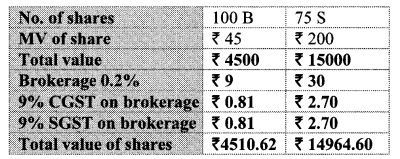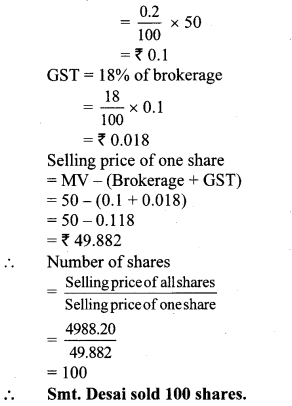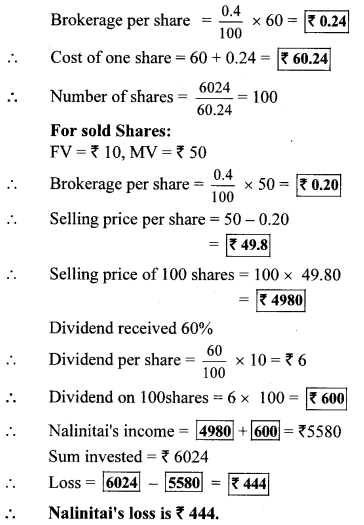# Maharashtra Board 10th Class Maths Part 1 Practice Set 4.4 Solutions Chapter 4 Financial Planning

Balbharti Maharashtra State Board Class 10 Maths Solutions covers the Practice Set 4.4 Algebra 10th Class Maths Part 1 Answers Solutions Chapter 4 Financial Planning.

## Practice Set 4.4 Algebra 10th Std Maths Part 1 Answers Chapter 4 Financial Planning

Question 1.
Market value of a share is ₹ 200. If the brokerage rate is 0.3% then find the purchase value of the share.
Solution:
Here, MV = ₹ 200, Brokerage = 0.3%
Brokerage = 0.3% of MV
= $$\frac { 0.3 }{ 100 }$$ × 200
= ₹ 0.6
∴ Purchase value of the share = MV + Brokerage
= 200 + 0.6
= ₹ 200.60
∴ Purchase value of the share is ₹ 200.60.

Question 2.
A share is sold for the market value of ₹ 1000. Brokerage is paid at the rate of 0.1%. What is the amount received after the sale?
Solution:
Here, MV = ₹ 1000, Brokerage = 0.1%
∴ Brokerage = 0.1 % of MV
= $$\frac { 0.1 }{ 100 }$$ × 1000
∴ Brokerage = ₹ 1
∴ Selling value of the share = MV – Brokerage
= 1000 – 1
= ₹ 999
∴ Amount received after the sale is ₹ 999.

Question 3.
Fill in the blanks given in the contract note of sale-purchase of shares.
(B – buy S – sell)Solution:
Here, Number of shares = 100,
MV of one share = ₹ 45
∴ Total value = 100 × 45
= ₹ 4500
Brokerage= 0.2% of total value 0.2
= $$\frac { 0.2 }{ 100 }$$ × 4500
CGST = 9% of brokerage
= $$\frac { 9 }{ 100 }$$ × 9 = ₹ 0.81
But, SGST = CGST
∴ SGST = ₹ 0.81
∴ Purchase value of shares
= Total value + Brokerage
= 4500 + 9 + 0.81 + 0.81
= ₹ 4510.62

ii. For selling shares:
Here, Number of shares = 75,
MV of one share = ₹ 200
∴ Total value = 75 × 200
= ₹ 15000
Brokerage = 0.2% of total value
= $$\frac { 0.2 }{ 100 }$$ × 15000
= ₹ 30
CGST = 9% of brokerage
= $$\frac { 9 }{ 100 }$$ × 30 = ₹ 2.70
But, SGST = CGST
∴ SGST = ₹ 2.70
∴ Selling value of shares = Total value – (Brokerage + CGST + SGST)
= 15000 – (30 + 2.70 + 2.70)
= 15000 – 35.40
= ₹ 14964.60Question 4.
Smt. Desai sold shares of face value ₹ 100 when the market value was ₹ 50 and received ₹ 4988.20. She paid brokerage 0.2% and GST on brokerage 18%, then how many shares did she sell?
Solution:
Here, face value of share = ₹ 100,
MV = ₹ 50,
Selling price of shares = ₹ 4988.20,
Rate of brokerage = 0.2%, Rate of GST = 18%
Brokerage = 0.2% of MVQuestion 5.
Mr. D’souza purchased 200 shares of FV ₹ 50 at a premium of ₹ 100. He received 50% dividend on the shares. After receiving the dividend he sold 100 shares at a discount of ₹ 10 and remaining shares were sold at a premium of ₹ 75. For each trade he paid the brokerage of ₹ 20. Find whether Mr. D’souza gained or incurred a loss? By how much?
Solution:
Here, FV = ₹ 50, Number of shares = 200,
MV of 1 share = FV + premium
= 50 + 100
= ₹ 150
∴ MV of 200 shares = 200 × 150 = ₹ 30,000
∴ Mr. D’souza invested amount
= MV of 200 shares + brokerage
= 30,000 + 20
= ₹ 30,020
For selling shares:
Rate of dividend = 50 %, FV = ₹ 50,
brokerage = ₹ 20
Number of shares = 200
Dividend per share = 50% of FV
= $$\frac { 50 }{ 100 }$$ × 50
= ₹ 25
∴ Dividend of 200 shares = 200 × 25 = ₹ 5,000
Now, 100 shares are sold at a discount of ₹ 10.
∴ Selling price of 1 share = FV – discount
= 50 – 10
= ₹ 40
∴ Selling price of 100 shares = 100 × 40
= ₹ 4000
∴ Amount obtained by selling 100 shares
= selling price – brokerage
= 4000 – 20
= ₹ 3980
Also, remaining 100 shares are sold at premium of ₹ 75.
∴ selling price of 1 share = FV + premium
= 50 + 75
= ₹ 125
∴ selling price of 100 shares = 100 × 125
= ₹ 12,500
∴ Amount obtained by selling 100 shares
= selling price – brokerage
= 12,500 – 20
= ₹ 12,480
∴ Mr D’souza income = 5000 + 3980 + 12480
= ₹ 21460
Now, Mr D’souza invested amount > income
∴ Mr D’souza incurred a loss.
∴ Loss = amount invested – income
= 30020 – 21460
= ₹ 8560
∴ Mr. D’souza incurred a loss of ₹ 8560.

Question 1.
Nalinitai invested ₹ 6024 in the shares of FV ₹ 10 when the Market Value was ₹ 60. She sold all the shares at MV of ₹ 50 after taking 60% dividend. She paid 0.4% brokerage at each stage of transactions. What was the total gain or loss in this transaction? (Textbook pg. no. 106)
Solution:
Rate of GST is not given in the example, so it is not considered.
For Purchased Shares:
FV = ₹ 10, MV = ₹ 60Question 2.
In the above example if GST was paid at 18% on brokerage, then the loss is ₹ 451.92. Verify whether you get the same answer. (Textbook pg, no. 107)
Solution:
For Purchased Shares:
FV = ₹ 10, MV = ₹ 60, sum invested = ₹ 6024, brokerage = 0.4 %, GST = 18%
Brokerage per share = $$\frac { 0.4 }{ 100 }$$ × 60 = ₹ 0.24 100
GST per share = $$\frac { 18 }{ 100 }$$ × 0.24 = ₹ 0.0432
∴ Cost of one share = 60 + 0.24 + 0.0432
= ₹ 60.2832
∴ Cost of 100 shares = 100 × 60.2832 = ₹ 6028.32
For sold shares:
FV = ₹ 10, MV = ₹ 50, brokerage = 0.4 %,
GST = 18%, Number of shares = 100
Brokerage per share = $$\frac { 0.4 }{ 100 }$$ × 50 = ₹ 0.20
GST per share = $$\frac { 18 }{ 100 }$$ × 0.20 = ₹ 0.036
Selling price per share = 50 – 0.2 – 0.036
= ₹ 49.764
Selling price of 100 shares = 100 × 49.764
= ₹ 4976.4
∴ Dividend per share = $$\frac { 60 }{ 100 }$$ × 10 = ₹ 6